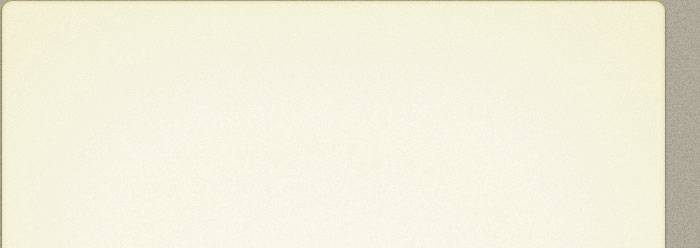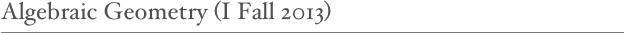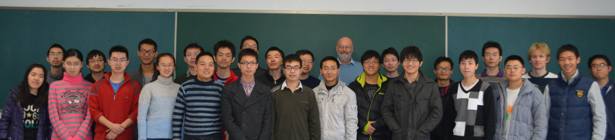EXAM

This is the take home exam and here is a set of worked solutions. You might take note of a comment by 姜清元 on Problem 2c. I have now also posted a revised edition of the notes.

A first characterization of Algebraic Geometry is: the study of the common zero sets of a collection of polynomial  equations in a given number of variables x1,...,xn. These polynomials were originally assumed to have real coefficients so that the zero set  would lie in real n-space. After the advent of complex numbers,  it was soon noticed that the theory became much more manageable if these coefficients were taken to be complex numbers (for instance a polynomial in a single real variable of degree d has at most d real solutions,  but if we take the coefficients complex, then the number of complex solutions is exactly d, if we are willing to count solutions with multiplicity). It had also been observed that things became even better if we include “solutions at infinity”. This means looking  for solutions in a somewhat bigger space then complex n-space, namely complex projective n-space. This was followed by the observation that  for much of the theory the only property of the complex numbers that intervened was that they make up an algebraically closed field. We thus arrive at the point of  what is called Projective Algebraic Geometry: the study of common zero sets of systems of homogeneous polynomials in n+1 variables x0,...,xn with coefficients in an algebraically closed field.

However, if it so happens that the polynomials have their coefficients in a smaller field that is not algebraically closed such as the field of rational numbers, then it makes sense (and there may be good reason) to ask for solutions with coefficients in that field. But this is often a subtle issue which usually involves Galois theory. Even when the field is that of the real numbers and this explains why it was not a good idea to start out that way. Things becomes even more complicated if the algebraically closed field is replaced by a ring, for instance the ring of integers. Such questions are by no means uninteresting, as many natural questions in number theory can be stated that way.  In the 1950s it was gradually recognized that if one is to accommodate this kind of generality, a complete rebuilding of the foundations  was called for. This foundational work was carried out during a relatively short period (1958-1970) under the leadership of A. Grothendieck. The tools and language developed by him (with his notion  of scheme taking the place of an algebraic variety) and the underlying way of looking at things are now universally accepted  as the framework to work in (and to formulate results about) algebraic geometry. At the same time, experience has taught us that the scheme setting is ill-suited for a first acquaintance with algebraic geometry, and this is why most of this course is concerned with Algebraic Geometry over an algebraically closed field.

Content of the course

We begin with developing the dictionary between geometry and algebra  in the most basic and elementary way: if k is an algebraically closed field, and A is a finitely generated k-algebra without nilpotent elements, then A can be understood as an algebra of k-valued functions on a topological space, the maximal ideal spectrum of A.  This leads us into algebraic geometry over the field k. Soon enough we will recognize the need to replace the maximal ideal spectrum by somewhat larger space, the prime ideal spectrum (=ordinary) spectrum. This may look strange at first, but turns out to do a much better job in geometrically representing algebraic properties. This then will lead us  naturally to the notion of a scheme. Along the way we consider some examples in a fair amount of detail. It is advised you get hold of the book  of Harthorne (see below).

Prerequisites

Basic commutative algebra (concerning rings and  modules) and a bit of Galois theory.

Literature

Apart from the Lecture notes (for an updated version go here), here is some literature that you might find useful to consult. For other opinions you may want to take a look at this mathoverflow question.

David Eisenbud and Joe Harris: The Geometry of Schemes, GTM 197. A good introduction to schemes and related notions.

Robin Hartshorne: Algebraic Geometry, Springer Verlag GTM 52. Still the most widely used introduction to modern algebraic geometry.

Liu Qing: Algebraic Geometry and Arithmetic curves Oxford Science Publications. The original motivation of the author was to give an exposition of arithmetic surfaces. But the first half of the book is an excellent introduction to schemes and the second half well illustrates the power of the scheme  approach.

David Mumford: The Red Book of Varieties and Schemes, Lecture Notes in Mathematics 1358. This is in fact two rather separate books which have been reprinted in a single volume. Relevant for this course is the part this (nowadays, yellow)  lecture note it is named after, which was essentially the first book on schemes meant for students. It is still a very good introduction, written in the author's characteristic style: informality paired with precision.

David Eisenbud: Commutative Algebra with a view toward Algebraic Geometry, GTM 150. A substantial text of about 780 pages. The topic of the subtitle  here enters mostly through local properties or via affine varieties. The book has detailed proofs, often accompanied by enlightening discussions. It shows that there is little difference between Commutative  Algebra and Local Algebraic Geometry.

Fu Lei: Algebraic Geometry,  a concise introduction (of about 260 p.) to the theory of schemes based on  a course taught at the Morningside Center. It is joint publication of Springer and Tsinghua UP and that is reflected by its price here on campus: for 39 it is a steal.

And for the brave:

Alexandre Grothendieck-Jean Dieudonné: Éléments de Géométrie Algébrique. Publications Mathématiques de l'IHES. This is the fundamental source. Only 4 chapters of the planned 13 have appeared, but they already comprise about 1500 pages. Go for it if you read French and want rigor and generality.

Johan de Jong et alii: The stacks project <http://stacks.math.columbia.edu>. This Wiki based enterprise is becoming the natural successor of EGA as the standard opus of reference for algebraic geometry. It is certainly as rigorous and general and goes well beyond the notion of a scheme. Many of the 75(!) chapters rest on only few of the preceding ones, so that often you can just start reading a chapter once you have already some basic knowledge of the field.

Ravi Vakil: MATH 216: Foundations of Algebraic Geometry. These course notes delve into the subject in a true Grothendieck spirit right from the start, yet do this in a way that makes prerequisites minimal. When you have finished working through the 700+ page manuscript you have also learned  a lot about category theory and homological algebra. It is on Vakil's website  available as a wordpress blog, which means that it cannot be accessed this side of the wall. I therefore put a pdf copy here.

Place and time

Tuesdays and Fridays 13:30-15:05 in Teaching Building 6, Sector A, Room  6A309, starting Sept. 17.

However the holiday arrangement entails that  the class on Sept. 20 (Fri) is moved to Sept. 22 (Sun)

(with the same time slot and place) and that classes on  Oct. 1 (Tue) and Oct. 4 (Fri) are canceled.

Material covered and exercises

Sept 17:  Finished the proof of  Lemma 2.2; Exerc. 4*, 8*, (9* has been scrapped).

Sept 22:  Finished with 2.13; Exerc. 10*, 11, 12, 13*, 14.

Sept 26: Finished Example 4.4; Exerc. 17*, 18.

Oct 8: Finished Example 4.12; Exerc. 19, 20*, 21, 22*.

Oct 11: Until Definition 6.2; Exerc. 23*, 27.

Oct 15: Until 6.8; Exerc. 28, 29*, 30*.

Oct 18: Until Proposition 7.3; Exerc. 31*, 32*, 33.

Oct 22: Finished Lemma 7.9; Exerc. 35*, 36*.

Oct 25: Until Corollary 8.6; Exerc. 38, 42*, 43*.

Oct 29: Until Lemma 9.6; Exerc. 44, 45*.

Nov 1: Until Theorem 9.12; Exerc. 46*.

Nov 5: Finished the universal derivation; Exerc. 47*, 48*.

Nov 8: Until Definition 10.4; Exerc. 49*, 50*.

Nov 12: Ch. 2 until 1.3; Exerc. 53*, 55*.

Nov 15: Completed section 2 of Ch. 2; Exerc. 57*, 58* (58a has been scrapped), 60.

Nov 19: Completed section 4 of Ch. 2; Exerc. 61, 62*, 63*.

Nov 22: Completed section 5 of Ch. 2;  Exerc. 64*, 65.

Nov 26: Finished proof of  7.1; Exerc. 66*.

Nov 29: Finished Corollary 8.5; Exerc. 67*, 68.

Dec 3: Until Proposition 8.11; Exerc. 69*, 70*, 71.

Dec 6: Until Lemma 9.5; Exerc. 72*.

Dec 10: Completed section 9; no exercises this time.

Dec 13: Until Bézout's theorem; Exerc. 73*.

Dec 17: Until Definition of a sheaf; Exerc. 75*, 76*.

Dec 20: Finished section 2 of Ch. 3; Exerc. 78*.

Dec 24: Finished section 3 of Ch. 3

(but go  here for the bigger picture)